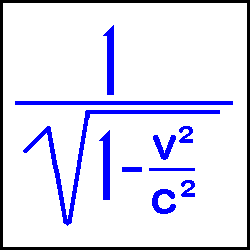Relativity CalculatorThe above formula is used for calculating the changes that occur when objects approach the speed of light. This was formulated by the German-American physicist and mathematician Albert Einstein (1879-1955) in his Special Theory of Relativity. Basically, an object in motion undergoes 3 relativistic changes: 1) An increase in mass 2) A contraction in the direction of travel (Lorentz Transformation) and 3) A "slowing down" of time. (Time Dilation)

Velocities in ordinary life which to us might seem incredibly fast have only a miniscule relativistic effect. For example, orbital velocity (5 miles per second) produces a relativistic factor of change of only 1.000000000360219.

Traveling at 93,141.1985 miles per second (half the speed of light) produces a factor of 1.1547005383792517. Here the velocity is incredibly fast and yet the change is still quite small.

 At .9 times the speed of light, the factor becomes 2.294157338705618. Finally, the effects of relativity become significant. What does this factor mean though? If you were in a spaceship traveling at .9 times the speed of light: 1) the ship's mass (and you) would increase by a factor of 2.294 2) the ship (and you) would contract in the direction of travel by 2.294, meaning a 300 foot ship would shrink to 130.77 feet. 3) Perhaps the most interesting change is that 1 year to you would seem to be 2.294 years for someone back on Earth.

Now for the calculator instructions. The first 2 buttons are self-explanatory. The third button is clicked when the number you entered in the input box is in terms of the speed of light.
The 4th button (the factor of change button) is clicked when you have input the relativistic factor and wish to determine the velocity required for this factor.

Input >>>>>